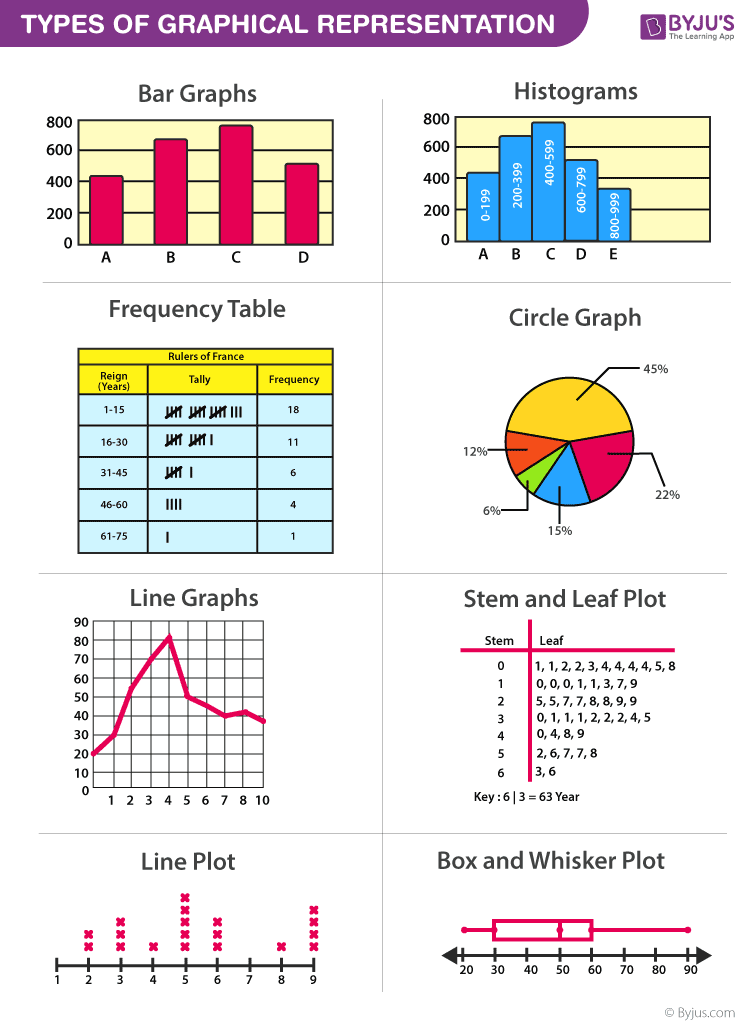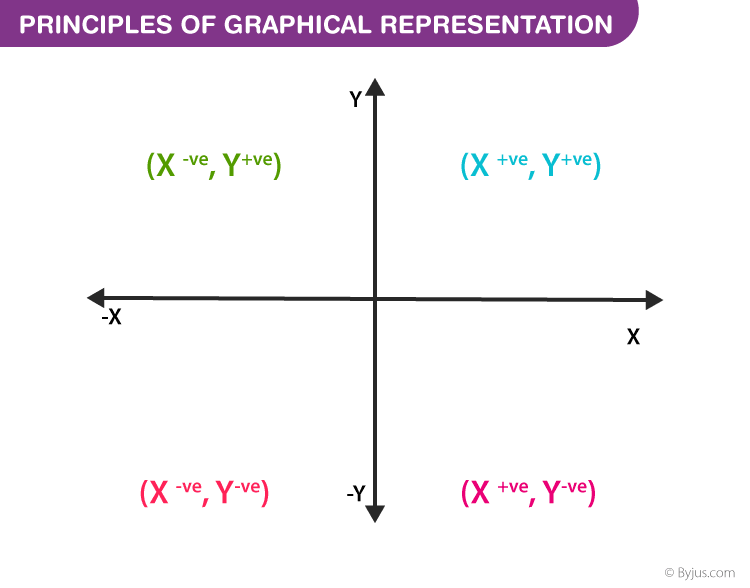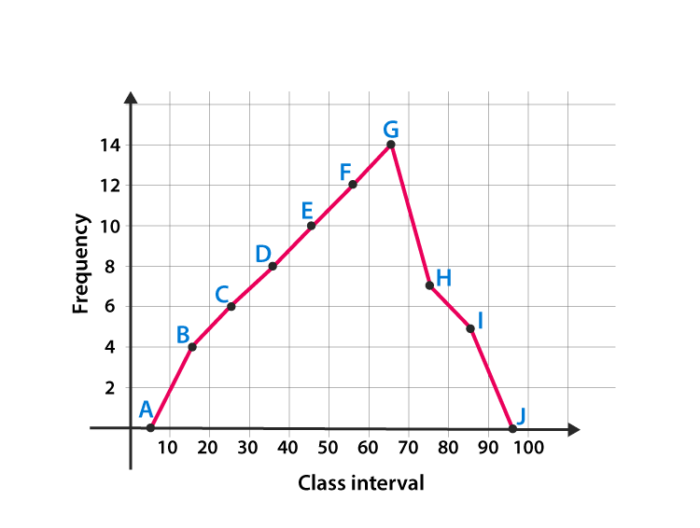Jet Set Go! All about Aeroplanes Jet Set Go! All about Aeroplanes

# Graphical Representation

Graphical Representation is a way of analysing numerical data. It exhibits the relation between data, ideas, information and concepts in a diagram. It is easy to understand and it is one of the most important learning strategies. It always depends on the type of information in a particular domain. There are different types of graphical representation. Some of them are as follows:

• Line Graphs – Line graph or the linear graph is used to display the continuous data and it is useful for predicting future events over time.
• Bar Graphs – Bar Graph is used to display the category of data and it compares the data using solid bars to represent the quantities.
• Histograms – The graph that uses bars to represent the frequency of numerical data that are organised into intervals. Since all the intervals are equal and continuous, all the bars have the same width.
• Line Plot – It shows the frequency of data on a given number line. ‘ x ‘ is placed above a number line each time when that data occurs again.
• Frequency Table – The table shows the number of pieces of data that falls within the given interval.
• Circle Graph – Also known as the pie chart that shows the relationships of the parts of the whole. The circle is considered with 100% and the categories occupied is represented with that specific percentage like 15%, 56%, etc.
• Stem and Leaf Plot – In the stem and leaf plot, the data are organised from least value to the greatest value. The digits of the least place values from the leaves and the next place value digit forms the stems.
• Box and Whisker Plot – The plot diagram summarises the data by dividing into four parts. Box and whisker show the range (spread) and the middle ( median) of the data.## General Rules for Graphical Representation of Data

There are certain rules to effectively present the information in the graphical representation. They are:

• Suitable Title: Make sure that the appropriate title is given to the graph which indicates the subject of the presentation.
• Measurement Unit: Mention the measurement unit in the graph.
• Proper Scale: To represent the data in an accurate manner, choose a proper scale.
• Data Sources: Include the source of information wherever it is necessary at the bottom of the graph.
• Keep it Simple: Construct a graph in an easy way that everyone can understand.
• Neat: Choose the correct size, fonts, colours etc in such a way that the graph should be a visual aid for the presentation of information.

## Graphical Representation in Maths

In Mathematics, a graph is defined as a chart with statistical data, which are represented in the form of curves or lines drawn across the coordinate point plotted on its surface. It helps to study the relationship between two variables where it helps to measure the change in the variable amount with respect to another variable within a given interval of time. It helps to study the series distribution and frequency distribution for a given problem. There are two types of graphs to visually depict the information. They are:

• Time Series Graphs – Example: Line Graph
• Frequency Distribution Graphs – Example: Frequency Polygon Graph

### Principles of Graphical Representation

Algebraic principles are applied to all types of graphical representation of data. In graphs, it is represented using two lines called coordinate axes. The horizontal axis is denoted as the x-axis and the vertical axis is denoted as the y-axis. The point at which two lines intersect is called an origin ‘O’. Consider x-axis, the distance from the origin to the right side will take a positive value and the distance from the origin to the left side will take a negative value. Similarly, for the y-axis, the points above the origin will take a positive value, and the points below the origin will a negative value.Generally, the frequency distribution is represented in four methods, namely

• Histogram
• Smoothed frequency graph
• Pie diagram
• Cumulative or ogive frequency graph
• Frequency Polygon

## Merits of Using Graphs

Some of the merits of using graphs are as follows:

• The graph is easily understood by everyone without any prior knowledge.
• It saves time
• It allows us to relate and compare the data for different time periods
• It is used in statistics to determine the mean, median and mode for different data, as well as in the interpolation and the extrapolation of data.

## Example for Frequency polygonGraph

Here are the steps to follow to find the frequency distribution of a frequency polygon and it is represented in a graphical way.

• Obtain the frequency distribution and find the midpoints of each class interval.
• Represent the midpoints along x-axis and frequencies along the y-axis.
• Plot the points corresponding to the frequency at each midpoint.
• Join these points, using lines in order.
• To complete the polygon, join the point at each end immediately to the lower or higher class marks on the x-axis.

### Question :

Draw the frequency polygon for the following data

 Class Interval 10-20 20-30 30-40 40-50 50-60 60-70 70-80 80-90 Frequency 4 6 8 10 12 14 7 5

### Solution :

Mark the class interval along x-axis and frequencies along the y-axis.

Let assume that class interval 0-10 with frequency zero and 90-100 with frequency zero.

Now calculate the midpoint of the class interval.

 Class Intervals Midpoints Frequency 0-10 5 0 10-20 15 4 20-30 25 6 30-40 35 8 40-50 45 10 50-60 55 12 60-70 65 14 70-80 75 7 80-90 85 5 90-100 95 0

Using the midpoint and the frequency value from the above table, plot the points A (5, 0), B (15, 4), C (25, 6), D (35, 8), E (45, 10), F (55, 12), G (65, 14), H (75, 7), I (85, 5) and J (95, 0).

To obtain the frequency polygon ABCDEFGHIJ, draw the line segments AB, BC, CD, DE, EF, FG, GH, HI, IJ, and connect all the points.### What are the Different Types of Graphical Representation?

Some of the various types of graphical representation include:

• Line Graphs
• Bar Graphs
• Histograms
• Line Plots
• Frequency Table
• Circle Graph, etc.

### What are the Advantages of Graphical Method?

Some of the advantages of graphical representation are:

• It makes data more easily understandable.
• It saves time.
• It makes the comparison of data more efficient.
Visit BYJU’S for more information on graphical representation and some other Maths-related articles, and also watch the videos to clarify the doubts.

 Related Links Circle Graph Formula Histogram Graphing Calculator Straight Lines